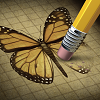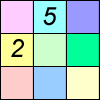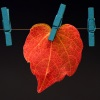# Search by Topic

#### Resources tagged with Resilient similar to Which Solids Can We Make?:

Filter by: Content type:
Age range:
Challenge level:

### There are 36 results

Broad Topics > Habits of Mind > Resilient### Triangles to Tetrahedra

##### Age 11 to 14 Challenge Level:

Imagine you have an unlimited number of four types of triangle. How many different tetrahedra can you make?### Tower of Hanoi

##### Age 11 to 14 Challenge Level:

The Tower of Hanoi is an ancient mathematical challenge. Working on the building blocks may help you to explain the patterns you notice.### Frogs

##### Age 11 to 14 Challenge Level:

How many moves does it take to swap over some red and blue frogs? Do you have a method?### Overlaps

##### Age 11 to 14 Challenge Level:

Can you find ways to put numbers in the overlaps so the rings have equal totals?### Can You Make 100?

##### Age 11 to 14 Challenge Level:

How many ways can you find to put in operation signs (+ - x ÷) to make 100?### Where Can We Visit?

##### Age 11 to 14 Challenge Level:

Charlie and Abi put a counter on 42. They wondered if they could visit all the other numbers on their 1-100 board, moving the counter using just these two operations: x2 and -5. What do you think?##### Age 11 to 14 Challenge Level:

By selecting digits for an addition grid, what targets can you make?### Cuboids

##### Age 11 to 14 Challenge Level:

Find a cuboid (with edges of integer values) that has a surface area of exactly 100 square units. Is there more than one? Can you find them all?### Estimating Angles

##### Age 7 to 14 Challenge Level:

How good are you at estimating angles?### Two and Two

##### Age 11 to 14 Challenge Level:

How many solutions can you find to this sum? Each of the different letters stands for a different number.### A Little Light Thinking

##### Age 14 to 16 Challenge Level:

Here is a machine with four coloured lights. Can you make two lights switch on at once? Three lights? All four lights?### Same Length

##### Age 11 to 16 Challenge Level:

Construct two equilateral triangles on a straight line. There are two lengths that look the same - can you prove it?### Elevenses

##### Age 11 to 14 Challenge Level:

How many pairs of numbers can you find that add up to a multiple of 11? Do you notice anything interesting about your results?### Wipeout

##### Age 11 to 14 Challenge Level:

Can you do a little mathematical detective work to figure out which number has been wiped out?### M, M and M

##### Age 11 to 14 Challenge Level:

If you are given the mean, median and mode of five positive whole numbers, can you find the numbers?### Isosceles Triangles

##### Age 11 to 14 Challenge Level:

Draw some isosceles triangles with an area of $9$cm$^2$ and a vertex at (20,20). If all the vertices must have whole number coordinates, how many is it possible to draw?### Nine Colours

##### Age 11 to 16 Challenge Level:

Can you use small coloured cubes to make a 3 by 3 by 3 cube so that each face of the bigger cube contains one of each colour?### Substitution Cipher

##### Age 11 to 14 Challenge Level:

Find the frequency distribution for ordinary English, and use it to help you crack the code.### Reflecting Squarely

##### Age 11 to 14 Challenge Level:

In how many ways can you fit all three pieces together to make shapes with line symmetry?### Odds and Evens

##### Age 11 to 14 Challenge Level:

Is this a fair game? How many ways are there of creating a fair game by adding odd and even numbers?### Reach 100

##### Age 7 to 14 Challenge Level:

Choose four different digits from 1-9 and put one in each box so that the resulting four two-digit numbers add to a total of 100.### Kite in a Square

##### Age 14 to 16 Challenge Level:

Can you make sense of the three methods to work out the area of the kite in the square?### Squares in Rectangles

##### Age 11 to 14 Challenge Level:

A 2 by 3 rectangle contains 8 squares and a 3 by 4 rectangle contains 20 squares. What size rectangle(s) contain(s) exactly 100 squares? Can you find them all?### Charlie's Delightful Machine

##### Age 11 to 16 Challenge Level:

Here is a machine with four coloured lights. Can you develop a strategy to work out the rules controlling each light?### Factors and Multiples Puzzle

##### Age 11 to 14 Challenge Level:

Using your knowledge of the properties of numbers, can you fill all the squares on the board?### Funny Factorisation

##### Age 11 to 14 Challenge Level:

Using the digits 1 to 9, the number 4396 can be written as the product of two numbers. Can you find the factors?### Product Sudoku

##### Age 11 to 14 Challenge Level:

The clues for this Sudoku are the product of the numbers in adjacent squares.### Difference Sudoku

##### Age 14 to 16 Challenge Level:

Use the differences to find the solution to this Sudoku.##### Age 11 to 14 Challenge Level:

What happens when you add a three digit number to its reverse?### Number Daisy

##### Age 11 to 14 Challenge Level:

Can you find six numbers to go in the Daisy from which you can make all the numbers from 1 to a number bigger than 25?### American Billions

##### Age 11 to 14 Challenge Level:

Play the divisibility game to create numbers in which the first two digits make a number divisible by 2, the first three digits make a number divisible by 3...### Factors and Multiples Game

##### Age 7 to 16 Challenge Level:

A game in which players take it in turns to choose a number. Can you block your opponent?### In a Box

##### Age 14 to 16 Challenge Level:

Chris and Jo put two red and four blue ribbons in a box. They each pick a ribbon from the box without looking. Jo wins if the two ribbons are the same colour. Is the game fair?### Data Matching

##### Age 14 to 18 Challenge Level:

Use your skill and judgement to match the sets of random data.### Number Lines in Disguise

##### Age 7 to 14 Challenge Level:

Some of the numbers have fallen off Becky's number line. Can you figure out what they were?